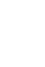# Maths

Head of Department: Michael Milner  (michael.milner@buxton.derbyshire.sch.uk )

### Key Stage 3

#### Course outline

Throughout Years 7, 8 and 9 students follow the National Curriculum for Maths. The level of difficulty for each area progresses throughout the three years, with higher ability students already starting to access GCSE level work. The broad topic areas covered are:

1 Number

• Adding, subtracting, multiplying and dividing
• Primes, factors and multiples
• Working with fractions
• Solving problems with percentages
• Rounding and place value

2 Algebra

• Simplifying expressions
• Expanding and factorising expressions
• Solving equations
• Drawing and working with graphs
• Sequences

3 Ratio and proportion

• Simplifying ratios
• Sharing in a ratio
• Solving ratio and proportion problems

4 Geometry and measures

• Areas of shapes
• Constructions
• Angle problems
• Pythagoras’ theorem
• Volume and surface area
• Transformations
• Units of measure

5 Probability

• Finding probabilities of events
• Listing outcomes

6 Statistics

• Finding averages
• Working with frequency tables
• Different types of charts and graphs
• Handling data

### Key Stage 4

The GCSE course in mathematics has a foundation tier (grades 1 – 5) and a higher tier (grades 4 – 9). Students’ prior performance will be used in deciding which course is most suitable for them.

The broad topic areas covered are:

1 Number

• Adding, subtracting, multiplying and dividing
• Primes, factors and multiples
• Working with fractions
• Solving problems with percentages

2 Algebra

• Simplifying expressions
• Expanding and factorising expressions
• Solving equations
• Drawing and working with graphs

3 Ratio and proportion

• Simplifying ratios
• Sharing in a ratio
• Solving ratio and proportion problems
• Direct and inverse proportion

4 Geometry and measures

• Areas of shapes
• Constructions and loci
• Angle problems
• Pythagoras’ theorem
• Trigonometry
• Circle theorems
• Volume and surface area

5 Probability

• Finding probabilities of events
• Listing outcomes
• Tree diagrams
• Conditional probability
• Venn diagrams

6 Statistics

• Finding averages
• Working with frequency tables
• Different types of charts and graphs
• Handling data
• Histograms
• Cumulative Frequency

We aim to inspire learners to develop confidence and fluency in mathematics, and to recognise the importance of mathematics in their own lives and society. We focus on enabling all learners to gain a deep understanding of mathematical concepts and to apply their skills and knowledge to solve problems in real-world situations.

Further details of the course content can be found on the AQA website.